MATLAB Function Referencefilter

Filter data with an infinite impulse response (IIR) or finite impulse response (FIR) filter

Syntax

• ```y = filter(b,a,X)
[y,zf] = filter(b,a,X)
[y,zf] = filter(b,a,X,zi)
y = filter(b,a,X,zi,dim)
[...] = filter(b,a,X,[],dim)
```

Description

The `filter` function filters a data sequence using a digital filter which works for both real and complex inputs. The filter is a direct form II transposed implementation of the standard difference equation (see "Algorithm").

```y = filter(b,a,X) ``` filters the data in vector `X` with the filter described by numerator coefficient vector `b` and denominator coefficient vector `a`. If `a(1)` is not equal to `1`, `filter` normalizes the filter coefficients by `a(1)`. If `a(1)` equals `0`, `filter` returns an error.

If `X` is a matrix, `filter` operates on the columns of `X`. If `X` is a multidimensional array, `filter` operates on the first nonsingleton dimension.

```[y,zf] = filter(b,a,X) ``` returns the final conditions, `zf`, of the filter delays. If `X` is a row or column vector, output `zf` is a column vector of `max(length(a),length(b))-1`. If `X` is a matrix, `zf` is an array of such vectors, one for each column of `X`, and similarly for multidimensional arrays.

```[y,zf] = filter(b,a,X,zi) ``` accepts initial conditions, `zi`, and returns the final conditions, `zf`, of the filter delays. Input `zi` is a vector of length `max(length(a),length(b))-1`, or an array with the leading dimension of size `max(length(a),length(b))-1` and with remaining dimensions matching those of `X`.

```y = filter(b,a,X,zi,dim) and [...] = filter(b,a,X,[],dim) ``` operate across the dimension `dim`.

Example

You can use `filter` to find a running average without using a `for` loop. This example finds the running average of a 16-element vector, using a window size of 5.

• ```data = [1:0.2:4]';
windowSize = 5;
filter(ones(1,windowSize)/windowSize,1,data)

ans =
0.2000
0.4400
0.7200
1.0400
1.4000
1.6000
1.8000
2.0000
2.2000
2.4000
2.6000
2.8000
3.0000
3.2000
3.4000
3.6000
```

Algorithm

The `filter` function is implemented as a direct form II transposed structure,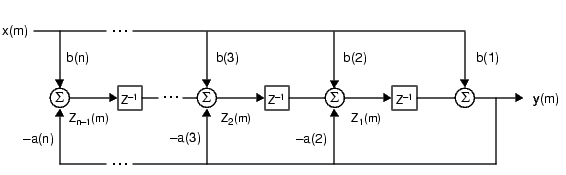or

• ```y(n) = b(1)*x(n) + b(2)*x(n-1) + ... + b(nb+1)*x(n-nb)
- a(2)*y(n-1) - ... - a(na+1)*y(n-na)
```

where `n-1` is the filter order, and which handles both FIR and IIR filters .

The operation of `filter` at sample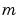is given by the time domain difference equations

•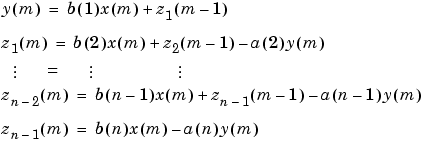The input-output description of this filtering operation in the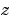-transform domain is a rational transfer function,

•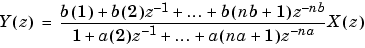See Also

`filter2`

`filtfilt`, `filtic` in the Signal Processing Toolbox

References

  Oppenheim, A. V. and R.W. Schafer. Discrete-Time Signal Processing, Englewood Cliffs, NJ: Prentice-Hall, 1989, pp. 311-312.

© 1994-2005 The MathWorks, Inc.